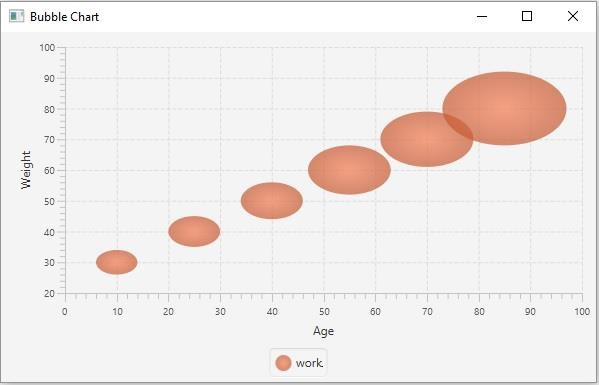# How to create a bubble chart using JavaFX?

The bubble chart accepts a series of data points (x, y) as input values and, creates bubbles for the data points in the given series. In JavaFX, you can create a bubble chart by instantiating the javafx.scene.chart.BubbleChart class.

Generally, in all X-Y charts, the data points plot two values (x, y). In the bubble chart, you can have an optional third value which is represented by the radius of the bubble.

While instantiating this class you must pass the two objects of the Axis class representing the x and y-axis (as parameters of the constructor). Since the Axis class is abstract you need to pass objects of its concrete subclasses, NumberAxis (for numerical values) or, CategoryAxis (String values).

Once you create the axes you can set labels to them using the setLabel() method.

## Setting data

The XYChart.Series represents the series of data items. You can create a series of points for the bubbles by instantiating this class. This class contains an observable list that holds all the points in the series.

The XYChart.Data represents a specific data point in the x-y plane. To create a point, you need to instantiate this class by passing the x and y values of the particular point.

Therefore, to create data for a bubble −

• Create a required number of points by instantiating the XYChart.Data class.

• Create a series by instantiating the XYChart.Series class.

• Get the observable list of the XYChart.Series class using the getData() method.

• Add the created data series to the area chart as −

bubbleChart.getData().add(series);

## Example

import javafx.application.Application;
import javafx.scene.Scene;
import javafx.scene.chart.BubbleChart;
import javafx.stage.Stage;
import javafx.scene.chart.NumberAxis;
import javafx.scene.chart.XYChart;
import javafx.scene.layout.StackPane;
public class BubbleChartExample extends Application {
public void start(Stage stage) {
//Creating X and Y axes
NumberAxis xAxis = new NumberAxis(0, 100, 10);
NumberAxis yAxis = new NumberAxis(20, 100, 10);
//Creating labels to the axes
xAxis.setLabel("Age");
yAxis.setLabel("Weight");
//Creating the Bubble chart
BubbleChart bubbleChart = new BubbleChart(xAxis, yAxis);
//Preparing data for bubble chart
XYChart.Series series = new XYChart.Series();
//Setting the data to bar chart
//Setting name to the bubble chart
series.setName("work");
//Creating a stack pane to hold the chart
StackPane pane = new StackPane(bubbleChart);
//Setting the Scene
Scene scene = new Scene(pane, 595, 350);
stage.setTitle("Bubble Chart");
stage.setScene(scene);
stage.show();
}
public static void main(String args[]){
launch(args);
}
}

## Output